# Block sparsity: An example from bio-chemical reaction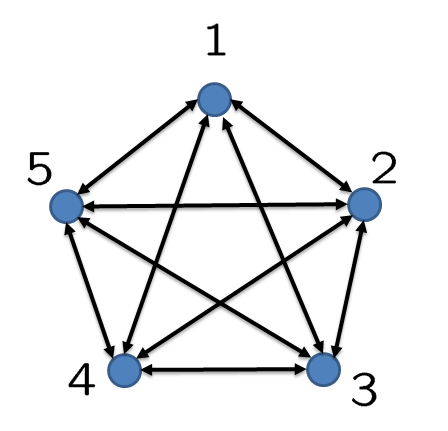Consider a network of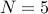systems coupled through the following dynamics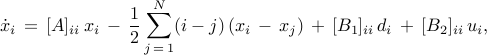where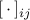denotes the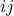th block of a matrix and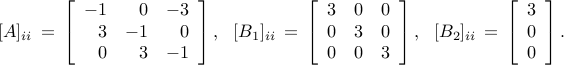Each system models a cyclic interconnection that arises in bio-chemical reactions (e.g., see Jovanovic et al. ’08). The performance weights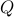and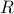are set to identity matrices.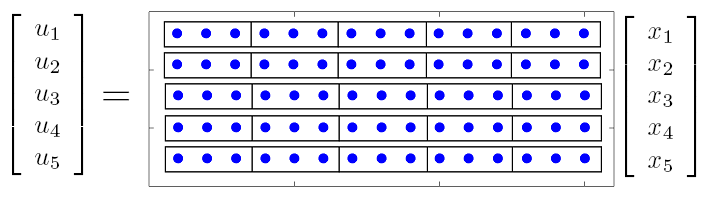Since each subsystem hascontrol input and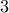states, the feedback gain matrix can be partitioned into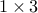submatrices as illustrated in the figure. We are interested in obtaining feedback gains with small number of block submatrices.

In this example, we use the weighted sum of Frobenius norms to design optimal block sparse feedback gains. To compare with elementwise sparsity, we also use the weighted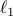norm for optimal sparse feedback design. In both cases, we set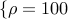,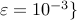and select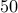logarithmically-spaced points for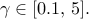We next show the Matlab code and the computational results obtained using lqrsp.m.

## Matlab code

```% Block sparsity example

% number of systems
N = 5;

% size of each system
nn = 3;
mm = 1;

% use cyclic condition to obtain unstable system
a = ones(1,nn);
b = 1.5*(sec(pi/nn))*a;

% state-space representation of each system
Aa = -diag(a) + diag(b(2:nn),-1);
Aa(1,nn) = -b(1);
Bb1 = diag(b);
Bb2 = zeros(nn,1);
Bb2(1) = b(1);

% non-symmetric weighted Laplacian matrix

Ad = toeplitz([1 0 0 1 0 0 1 0 0 1 0 0 1 0 0]);
for i = 1 : N
for j = 1 : N
if i ~= j
cij = 0.5 * ( i - j );
else
cij = 0;
end
Ad( nn*(i-1)+1 : nn*i, nn*(j-1)+1 : nn*j) = cij * eye(nn);
end
end

% take the sum of each row

% form the Laplacian matrix

% state-space representation of the interconnected system

A  = kron(eye(N), Aa) - L;
B1 = kron(eye(N), Bb1);
B2 = kron(eye(N), Bb2);
Q  = eye(nn*N);
R  = eye(N);

% compute block sparse feedback gains
options_blkwl1 = struct('method','blkwl1','gamval', ...
logspace(-1,log10(5),50),'rho',100,'maxiter',1000,'blksize',[1 3], ...
'reweightedIter',1);

tic
solpath_blkwl1 = lqrsp(A,B1,B2,Q,R,options_blkwl1);
toc

% compute element sparse feedback gains
options_wl1 = struct('method','wl1','gamval', ...
logspace(-1,log10(5),50),'rho',100,'maxiter',1000,'blksize',[1 1], ...
'reweightedIter',1);

tic
solpath_wl1 = lqrsp(A,B1,B2,Q,R,options_wl1);
toc
```

## Computational results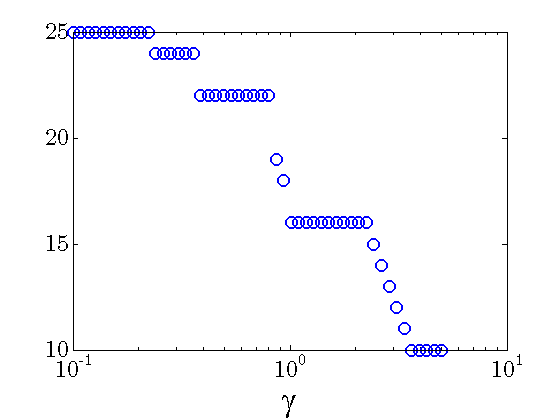Number of nonzero block submatrices decreases with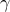.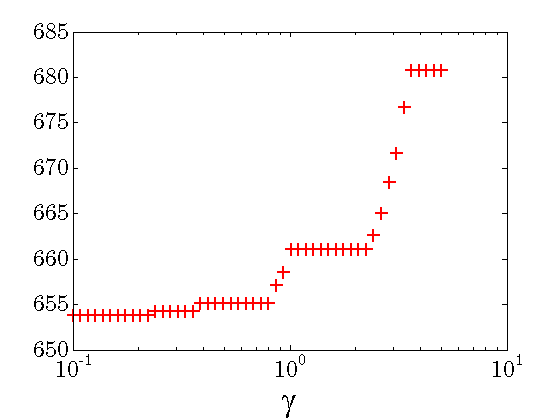Quadratic performance deteriorates with.
The following example illustrates two feedback gains resulting from the block sparse and sparse feedback designs. These feedback gains have close quadratic performance,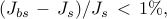and the same number of nonzero elements, but different number of nonzero block submatrices. Furthermore, as illustrated below, the optimal sparse feedback gain requires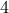more communication links than the optimal block sparse feedback gain.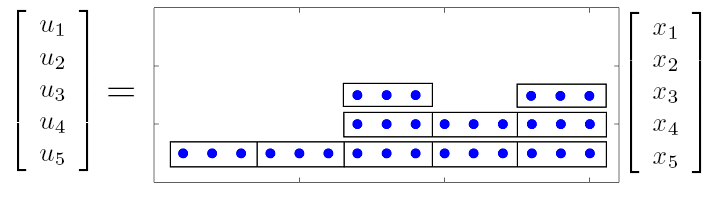Structure of the optimal block sparse feedback gain. The algorithm with the weighted sum of Frobenius norms and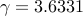yields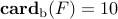(black boxes) and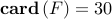(blue dots). Note that the first two rows are identically equal to zero (indicated by blank space). This implies that the subsystemsand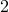do not need to be actuated.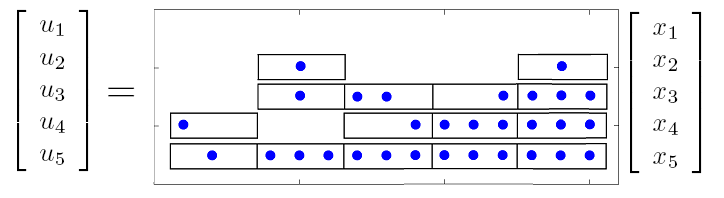Structure of the optimal sparse feedback gain. The algorithm with the weightednorm and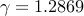yields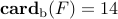(black boxes) and(blue dots).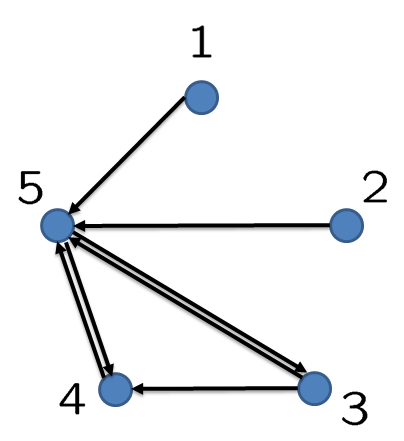Communication graph of the optimal block sparse feedback gain.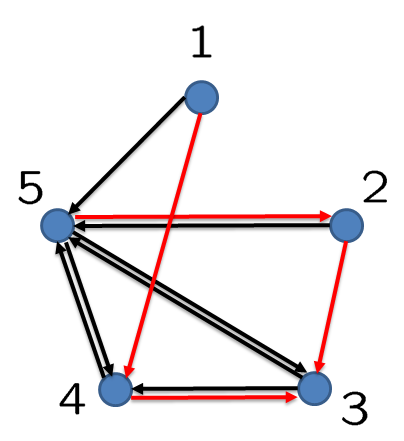Communication graph of the optimal sparse feedback gain. Red color highlights the additional links (relative to the optimal block sparse feedback gain).